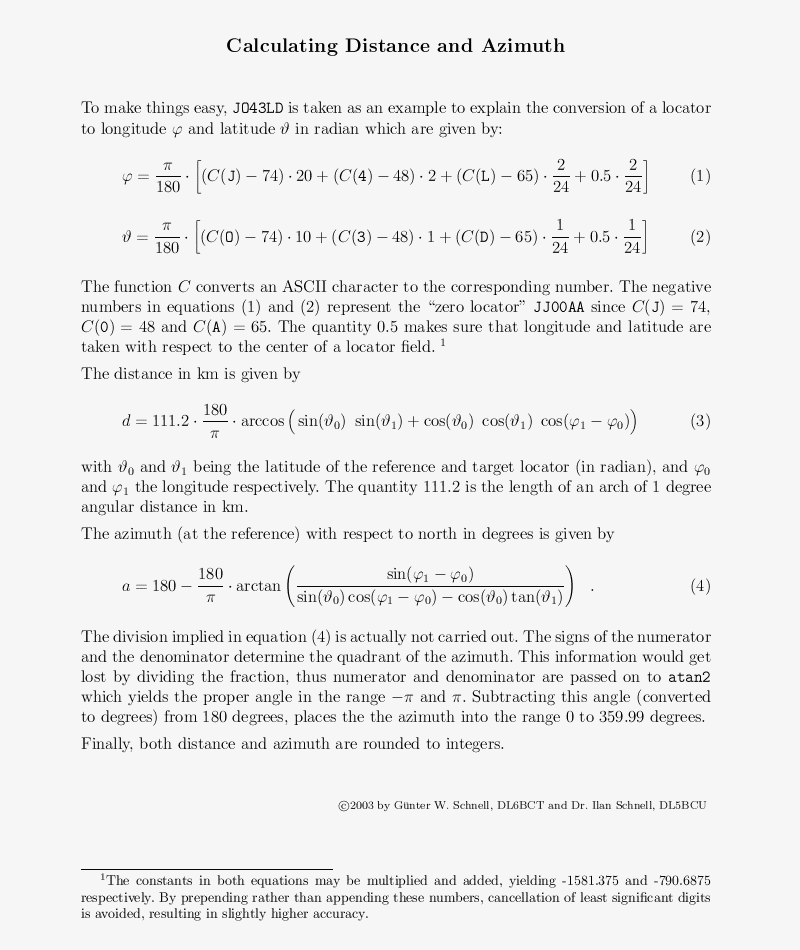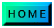### Distance and Azimuth

 Locator 1: Locator 2:
 Distance: Azimuth:
How to use the online calculator:
Enter one or two locators and press the Calculate button. The calculator will show the respective latitude and longitude.
If you enter two locators, it will also show distance and azimuth seen from the reference locator 1 to the target locator 2.
If you enter an uncomplete locator, e.g. JO43 or only JO, calculations are carried out with respect to the middle of this larger square.

The PHP script for this calulator was written by Dipl.-Phys. Arvin Schnell and Dipl.-Ing. Günter W. Schnell, using the equations discussed below.

a discussion of the equations used to calculate distance and azimuth of a target locator with respect to a reference locator (also availabe as PDF document math.pdf).
a Perl script implementing these equations and a short example.Perl Script

```#!/usr/bin/perl -w

use strict;
use Math::Trig;

my @loc;

\$loc = uc "JO43LD";				# Replace with your own Locator!

print "\nReference locator is \$loc\n\nEnter: ";

while (<>) {

chomp; s/\s+//g;

exit if /^exit\$|^quit\$/;

if    (/help/) { &help }
elsif (/-r([A-R]{2}\d\d[A-X]{2})/i) { \$loc = uc \$1; print "\nReference locator is \$loc\n\nEnter: "; next }
elsif (/([A-R]{2}\d\d[A-X]{2})/i)   { \$loc = uc \$1; &doit }
elsif (/(\d\d[A-X]{2})/i)           { \$loc = uc substr (\$loc, 0, 2).\$1; &doit }
else                                { print "\nNot a valid locator!\n" }

print "\nEnter: ";

}

sub doit {

my (@lng, @lat);

for (0..1) {

my @pos = unpack 'C6', \$loc[\$_];

\$lng[\$_] = deg2rad (-1581.375 + \$pos * 20 + \$pos * 2 + \$pos/12);
\$lat[\$_] = deg2rad (-790.6875 + \$pos * 10 + \$pos     + \$pos/24);

}

my \$tmp = sin (\$lat) * sin (\$lat) + cos (\$lat) * cos (\$lat) * cos (\$lng - \$lng);

my \$dist = int (111.2 * rad2deg (acos (\$tmp < 1 ? \$tmp : 1)) + 0.5);

\$tmp = atan2 (sin (\$lng - \$lng),
sin (\$lat) * cos (\$lng - \$lng) - cos (\$lat) * tan (\$lat));

my \$azim = \$dist ? int (180 - rad2deg (\$tmp) + 0.5) : 0;

printf "\n  Distance from %s to %s is %s km\n  Azimuth seen from %s is %s deg\n", @loc, \$dist, \$loc, \$azim;

}

sub help {

my \$help = <<HELP;

[target locator] (4 or 6 digits)
calculates distance and azimuth of target
locator with respect to reference locator
(currently \$loc). If prefix is omitted,
the current reference locator\'s prefix is
inserted.

[<-r> reference locator] (6 digits)
temporarily redefines reference locator.

Redefine reference locator permanently by
editing line 8.

[<help>]
shows this text.

[<exit> or <quit>]
exits this program.

HELP

for (\$help) { s|<|\033[1m|go; s|>|\033[m|go }

print \$help;
}

# Author: Wolf, DL6BCT (Jan 2002)
```

Example: Calculate distance and azimuth from JO43LD to IO87UJ, seen from JO43LD.

```Reference locator is JO43LD

Enter: io87uj

Distance from JO43LD to IO87UJ is 854 km
Azimuth seen from JO43LD is 308 deg

Enter:
```